# Lesson Tutor: Teaching or learning about multiplying 2 by 3 digits.

LINE DANCING: Multiplying 2 by 3 (or more!) digits
Joanne Mikola

This example taken from the print ready worksheet found at  https://www.lessontutor.com/jmmath/

367
x 24

First off – always write these ‘big’ multiplication questions like the example (up and down), NOT like this: 367 x 24 (side by side). Sometimes this will mean doing the figuring in the margin of your worksheet page. One of my most fondly remembered math teachers had us draw a 2″ inch line down the right hand side of every page of paper we used for math – just for this purpose. Alternatively, you could use a separate piece of paper. If it is for a test, be sure that all of your workings are well labeled, attached and submitted.

Secondly – If you have a choice (and you usually will), always put the bigger, or longer, number on the upper line. We both know that it does not matter the order in which you choose to work – anything times something will give you the same result as something times anything.

Thirdly – as long as you know your multiplication tables from zero to 9, you’ll never have any trouble with these sorts of questions – regardless of how many digits are in either one. Here’s how to do it. Step by step and line by line. Line dancing made easy.

Step 1: On the line directly below the question, work from the right hand side to the left, and make little bitty notes as you go. I would be thinking and saying in my head the following as I worked…
“4 multiplied by 7 is 28. 8 goes below in the ‘ones’ column, carry the 2”. It would look like this in my workbook: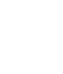Step 2: Multiply the 4 by the number in the ‘tens’ column – in this case the 6.
4 times 6 is 24. Add the 2 (carried over from the ‘ones’ column before) place the ‘6’ of 26 to the left of the 8, below. Carry another 2 over.Step 3: 4 times 3 is twelve. Add the 2 carried over to make 14. Write the 14 in front of everything since there are no other numbers to work with for the moment.Step 4: We’ll now multiply the entire top number (367) by the 2 in the tens column below, using the same right to left procedure including carry overs. But we have completely finished working in the ‘ones’ column so we’ll shift our inserted answers over one spot. You could put a mark of some description under the 8 in the answer to block it off, but practice will have you doing the shift automatically. It will look like a staircase when you are done.
2 times 7 is 14. Write the 4 in the tens column directly under the 6. Carry the one (1) to the hundreds column.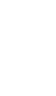Step 5: 2 times 6 is 12. Add 1 is 13. Print the 3 under the 4 in the hundreds column and carry the one (1) to the thousands column.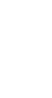Step 6: 2 times 3 is 6, add the one to equal 7. Place this in the thousands column.  Draw a line under the entire number.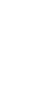Step 7: You’re almost done! Just easy stuff left – add the colorful 2 rows of numbers, ignoring the ones from the original question. That means the 1468 and the 734* remember – there is always NOTHING in the second row ones column.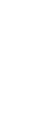If you are going on to multiply any number of numbers by larger ones, keep dancing one step to the left for every number you are multiplying by. It will never matter if these numbers have 2 or twenty columns, it will be done the same way every time. Here’s a picture to show you the stair pattern I was telling you about earlier. Even if those numbers have decimals!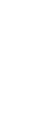Now go ahead and print the worksheet at  https://www.lessontutor.com/jmmath/ and play away! Colours not necessary but use them if it helps you to see the columns more clearly